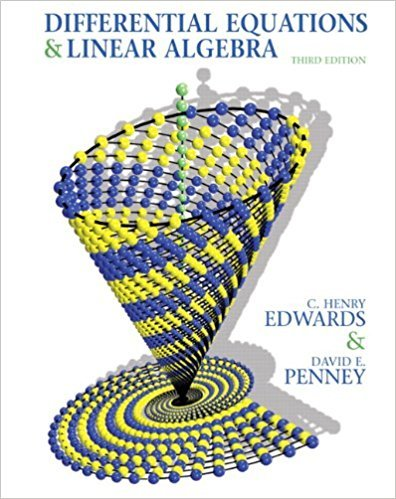×
×

# Solutions for Chapter 3.2: Differential Equations and Linear Algebra 3rd Edition## Full solutions for Differential Equations and Linear Algebra | 3rd Edition

ISBN: 9780136054252Solutions for Chapter 3.2

Solutions for Chapter 3.2
4 5 0 254 Reviews
18
4
##### ISBN: 9780136054252

Since 30 problems in chapter 3.2 have been answered, more than 11471 students have viewed full step-by-step solutions from this chapter. Differential Equations and Linear Algebra was written by and is associated to the ISBN: 9780136054252. This textbook survival guide was created for the textbook: Differential Equations and Linear Algebra, edition: 3. This expansive textbook survival guide covers the following chapters and their solutions. Chapter 3.2 includes 30 full step-by-step solutions.

Key Math Terms and definitions covered in this textbook
• Affine transformation

Tv = Av + Vo = linear transformation plus shift.

• Block matrix.

A matrix can be partitioned into matrix blocks, by cuts between rows and/or between columns. Block multiplication ofAB is allowed if the block shapes permit.

• Characteristic equation det(A - AI) = O.

The n roots are the eigenvalues of A.

• Cross product u xv in R3:

Vector perpendicular to u and v, length Ilullllvlll sin el = area of parallelogram, u x v = "determinant" of [i j k; UI U2 U3; VI V2 V3].

• Eigenvalue A and eigenvector x.

Ax = AX with x#-O so det(A - AI) = o.

• Free variable Xi.

Column i has no pivot in elimination. We can give the n - r free variables any values, then Ax = b determines the r pivot variables (if solvable!).

• Full column rank r = n.

Independent columns, N(A) = {O}, no free variables.

• Least squares solution X.

The vector x that minimizes the error lie 112 solves AT Ax = ATb. Then e = b - Ax is orthogonal to all columns of A.

• Left nullspace N (AT).

Nullspace of AT = "left nullspace" of A because y T A = OT.

• Normal equation AT Ax = ATb.

Gives the least squares solution to Ax = b if A has full rank n (independent columns). The equation says that (columns of A)·(b - Ax) = o.

• Normal matrix.

If N NT = NT N, then N has orthonormal (complex) eigenvectors.

• Polar decomposition A = Q H.

Orthogonal Q times positive (semi)definite H.

• Projection matrix P onto subspace S.

Projection p = P b is the closest point to b in S, error e = b - Pb is perpendicularto S. p 2 = P = pT, eigenvalues are 1 or 0, eigenvectors are in S or S...L. If columns of A = basis for S then P = A (AT A) -1 AT.

• Singular Value Decomposition

(SVD) A = U:E VT = (orthogonal) ( diag)( orthogonal) First r columns of U and V are orthonormal bases of C (A) and C (AT), AVi = O'iUi with singular value O'i > O. Last columns are orthonormal bases of nullspaces.

• Solvable system Ax = b.

The right side b is in the column space of A.

• Spectral Theorem A = QAQT.

Real symmetric A has real A'S and orthonormal q's.

• Spectrum of A = the set of eigenvalues {A I, ... , An}.

Spectral radius = max of IAi I.

• Stiffness matrix

If x gives the movements of the nodes, K x gives the internal forces. K = ATe A where C has spring constants from Hooke's Law and Ax = stretching.

• Unitary matrix UH = U T = U-I.

Orthonormal columns (complex analog of Q).

• Volume of box.

The rows (or the columns) of A generate a box with volume I det(A) I.

×